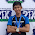## Circuits Frequency Response Objective Questions:

 Consider the parallel RLC circuit having R= 1, L= 1H, C=1F . What type of response will the circuit produce?
A. Under damped
B. Over damped
C. Critically damped
D. None of these

 How much inductance is needed to resonate at 5 kHz with a capacitance of 12nF?
A. 2652 H
B. 11.844 H
C. 3.333 H
D. 84.33 mH

 The difference between the half power frequencies is called the
A. Quality factor
B. Resonant frequency
C. Bandwidth
D. Cutoff frequency

 A parallel RLC circuit has C=0.25F & L=2H. The value of R which will create unity damping factor is
A. 1
B. 2
C. 0.5
D. 4

 A zero of the transfer function
10(s+1)
H(s) =  ------------
(s+2)(s+3)
is at
A. 10
B. -1
C. -2
D. -3

 On the Bode magnitude plot, the slope of the pole 1/(5 + jω)is
A. 20 dB/decade
B. 40 dB/decade
C. - 40 dB/decade
D. -20 dB/decade

 On the bode phase plot, the slope of [1 + j10ω - ω2/25]2 is
A. 45°/decade
B. 90°/decade
C. 135°/decade
D. 180°/decade

 In an electric circuit, the dual of resistance is
A. Conductance
B. Capacitance
C. Open circuit
D. Inductance

 In a series RLC circuit, which of these quality factors has the steepest curve at resonance?
A. Q = 20
B. Q = 12
C. Q = 8
D. Q = 4

 In a parallel RLC circuit, the bandwidth B is directly proportional to R
A. True
B. False

9:07 PM

#### 2 comments:

1.Hi. Shouldn't the answer to question 9 be (A) since for series resonance, a steep curve (i.e. narrow bandwidth) implies high quality factor?
**Alexander, C. and Sadiku M. Fundamentals of Electric Circuits, 5th ed.page 664, question 14.6.

1.yes 9 A is right answer